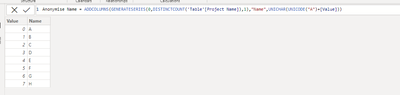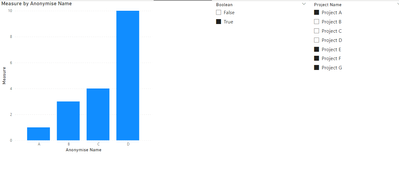cancel
Showing results for
Did you mean:Frequent Visitor

## Anonymize names and assign Letters

Hi,

I've been stuck on the following problem and hoping someone can help me on this.

I have a data table which has the following columns:

Project Name - String

Anonymise - Boolean

Location - String

Area - Number

using a slicer, the user is able to click on the project name and then select yes or no if that name should be anonymised.

I would like to anonymise the project name and then sort the selection according to area to assign a chronological letter.

Eg.

This is the data table

 Project Name Area Project A 10 Project B 23 Project C 43 Project D 5 Project E 1 Project F 3 Project G 4

I have selected Project A, E , F, G for a bar chart and have selected the names are anonomised, therefore the output I'm looking for isIs this possible?

Any advice would be much appricated.

1 ACCEPTED SOLUTIONCommunity Support

Hi @Fali324 ,

First create a new table:

``Anonymise Name = ADDCOLUMNS(GENERATESERIES(0,DISTINCTCOUNT('Table'[Project Name]),1),"Name",UNICHAR(UNICODE("A")+[Value]))``

Output:Then create a field parameter:Add a new column to the parameter table:

``Boolean = IF([Parameter Order]=0,"False","True")``

Output:Create a slicer:Apply the measure to the column chart:

``````Measure =
var _b = CALCULATE(SUM('Table'[Area]),FILTER(_a,[Index]=SELECTEDVALUE('Anonymise Name'[Value])+1))
var _c = SUM('Table'[Area])
return
IF(SELECTEDVALUE(Parameter[Boolean])="True"&&ISFILTERED(Parameter[Boolean]),_b,_c)``````Final output:Best Regards,

Jianbo Li

If this post helps, then please consider Accept it as the solution to help the other members find it more quickly.Community Support

Hi @Fali324 ,

First create a new table:

``Anonymise Name = ADDCOLUMNS(GENERATESERIES(0,DISTINCTCOUNT('Table'[Project Name]),1),"Name",UNICHAR(UNICODE("A")+[Value]))``

Output:Then create a field parameter:Add a new column to the parameter table:

``Boolean = IF([Parameter Order]=0,"False","True")``

Output:Create a slicer:Apply the measure to the column chart:

``````Measure =
var _b = CALCULATE(SUM('Table'[Area]),FILTER(_a,[Index]=SELECTEDVALUE('Anonymise Name'[Value])+1))
var _c = SUM('Table'[Area])
return
IF(SELECTEDVALUE(Parameter[Boolean])="True"&&ISFILTERED(Parameter[Boolean]),_b,_c)``````Final output:Best Regards,

Jianbo Li

If this post helps, then please consider Accept it as the solution to help the other members find it more quickly.